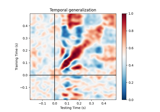# mne.decoding.PSDEstimator¶

class mne.decoding.PSDEstimator(sfreq=6.283185307179586, fmin=0, fmax=inf, bandwidth=None, adaptive=False, low_bias=True, n_jobs=1, normalization='length', verbose=None)[source]

Compute power spectral density (PSD) using a multi-taper method.

Parameters
sfreqfloat

The sampling frequency.

fminfloat

The lower frequency of interest.

fmaxfloat

The upper frequency of interest.

bandwidthfloat

The bandwidth of the multi taper windowing function in Hz.

Use adaptive weights to combine the tapered spectra into PSD (slow, use n_jobs >> 1 to speed up computation).

low_biasbool

Only use tapers with more than 90% spectral concentration within bandwidth.

n_jobsint

Number of parallel jobs to use (only used if adaptive=True).

normalizationstr

Either “full” or “length” (default). If “full”, the PSD will be normalized by the sampling rate as well as the length of the signal (as in nitime).

verbose

If not None, override default verbose level (see mne.verbose() and Logging documentation for more). If used, it should be passed as a keyword-argument only.

Methods

 __hash__(/) Return hash(self). fit(epochs_data, y) Compute power spectral density (PSD) using a multi-taper method. fit_transform(X[, y]) Fit to data, then transform it. transform(epochs_data) Compute power spectral density (PSD) using a multi-taper method.
fit(epochs_data, y)[source]

Compute power spectral density (PSD) using a multi-taper method.

Parameters
epochs_dataarray, shape (n_epochs, n_channels, n_times)

The data.

yarray, shape (n_epochs,)

The label for each epoch.

Returns
selfinstance of PSDEstimator

The modified instance.

fit_transform(X, y=None, **fit_params)[source]

Fit to data, then transform it.

Fits transformer to X and y with optional parameters fit_params and returns a transformed version of X.

Parameters
Xarray, shape (n_samples, n_features)

Training set.

yarray, shape (n_samples,)

Target values.

**fit_paramsdict

Additional fitting parameters passed to self.fit.

Returns
X_newarray, shape (n_samples, n_features_new)

Transformed array.

transform(epochs_data)[source]

Compute power spectral density (PSD) using a multi-taper method.

Parameters
epochs_dataarray, shape (n_epochs, n_channels, n_times)

The data.

Returns
psdarray, shape (n_signals, n_freqs) or (n_freqs,)

The computed PSD.

## Examples using mne.decoding.PSDEstimator¶